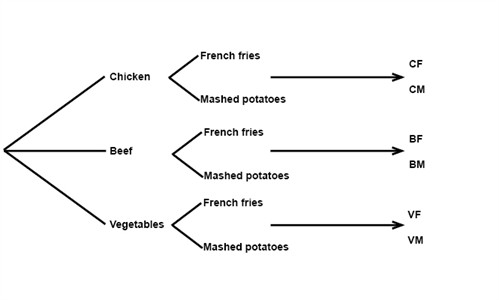# Calculating the outcome

To solve problems where the solution symbolizes a number of possible combinations i.e. outcomes, we can use a tree-diagram.

Example

Planet Bollywood offers chicken (C), beef (B) or vegetables (V) served with either French fries (F) or mashed potatoes (M). How many possible dinner alternatives or possible outcomes are there at Planet Bollywood.

We draw a tree-diagramThe total number of possible outcomes are 6, 3 ∙ 2 = 6. This principle is called the fundamental counting principle and the rule is as follows.

If event x (in this case the chicken, the beef and the vegetables) can occur in x ways. And event y (in this case French fries or mashed potatoes) can occur in y ways. The x followed by y can occur in x ∙ y ways.

## Video lesson

How many outcomes are possible when a die is rolled three times?